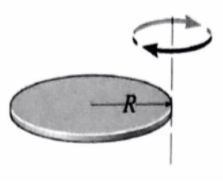# Problem: The moment of inertia of a disk with an axis through center of mass and perpendicular to the disk is, I = 1/3 MR2. Use the parallel axis theorem to find the rotational inertia for a disk that is rotating about an axis through the edge of the disk and perpendicular to the disk.

###### FREE Expert Solution

From the parallel axis theorem, the moment of inertia of an object about an axis through its center of mass is the minimum moment of inertia for an axis in that direction in space.

92% (30 ratings)###### Problem Details

The moment of inertia of a disk with an axis through center of mass and perpendicular to the disk is, I = 1/3 MR2. Use the parallel axis theorem to find the rotational inertia for a disk that is rotating about an axis through the edge of the disk and perpendicular to the disk.